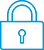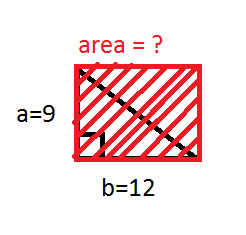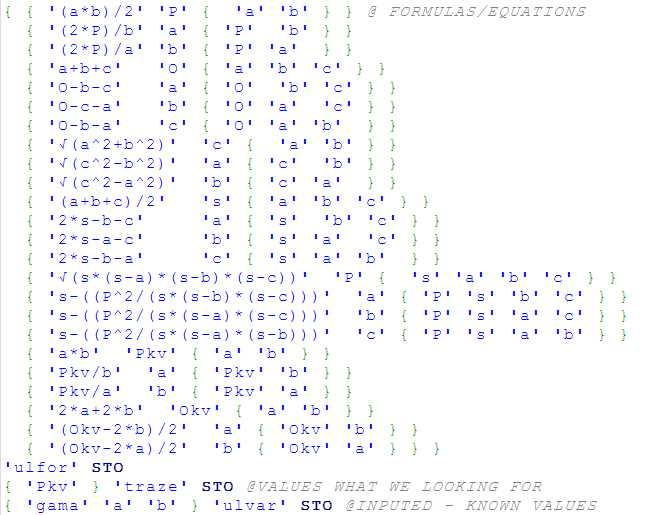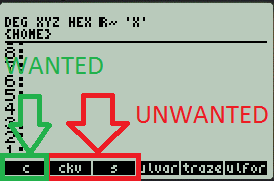cancel
Showing results for
Search instead for
Did you mean:It has been a while since anyone has replied. Simply ask a new question if you would like to start the discussion again.Level 4
138 92 2 5
Message 1 of 2
385
Flag Post

# Please help me with this program if you can ... made for HP 50g graphing calculatorHP Recommended

Hello dear HP Community ,

So , first of all , i have specific task for all members and those who's not right now but they planned to be as well

I'm working on one program for a while of time but now I'm stuck with some things inside of program or function or whatever you want to call that. Program is intended to HP 50g graphing calculator.

First of all , I must describe to you what I'm attending to do with my program.

So , my intention is to make a program ( HP 50g graphing calculator ) able to 'solve mathematical problems' itself , but not every mathematical problem and not especially "itself" because this is not any AI to make a work an own. I hope that you understand a task.

If you did not understand that what i wrote before , I have some detailed explanation for you ... so let suppose that I'm making a program which have ability to found a solution for specific math task after you , as an 'user' , define all elements including written formulas and other notations like input variables , variables what you search for and others inside of that task.

So , what I'm trying to do is make a program which will be able to read input variables and search for other variables which was not shown before and these variables need to be calculated or eventually evaluated by using input equations ( formulas )  or some other procedures. I initially referred on functions that were easier to count with like linear functions

So lets make a mathematical task. Let suppose that I'm dealing with a simple task from planar geometry.

So , I have a triangle which have a known sides a and b , and known angle between those sides - let suppose that

angle is called gama. In order to work with real numbers i used for side a value of 9 , for side b value od 12 and for angle between these two sides 90°00'00" ( rectangle ) .

So , my task is how to get area of rectangle which carries those right triangle ( because of right angle of 90° )

I will draw this task described above:this is my task with known angle , sides a and b

Now this is very easy task , yeah I know , but let's suppose that you must find other elements of triangle such as remaining two angles and side c ( diagonal of this triangle ) and suppose that triangle and generally some other task which is much

harder to solve and which have more steps of solving than this one .

REAL IMPORTANT NOTE - this is only an example of problem , I must work on program which will able to resolve any task which contains multiple computations like ... I don't know ... task which have MULTIPLE COMPUTATIONS !!!

So , I made some program which have a unique way for solving these or maybe harder tasks instead of this one.

So let's suppose that we also have information about how to calculate area of triangle , how to calculate perimeter of triangle and so forth...

Functioning on this program relies on lists . So i mean next ,

I have a list which containes other smaller list ( list of lists ) and in each of that list i have elements which describe how each formula ( equation ) work... to be explicit I mean that i have formula which is written in the way that HP 50g graphing calculator can understand what i wrote , and i got  input parameters ( list of global variables names ) which is necessary for correct functioning of each  formula ( equation ) and also i get name of variable which which represent what i calculated on that single formula.

So i give you an example , if I'm calculating a different elements of triangle I use this list of lists ...

ƒ - is sign for square root of number

{ { '(a*b)/2' 'P' { 'a' 'b' } }
{ '(2*P)/b' 'a' { 'P' 'b' } }
{ '(2*P)/a' 'b' { 'P' 'a' } }
{ 'a+b+c' 'O' { 'a' 'b' 'c' } }
{ 'O-b-c' 'a' { 'O' 'b' 'c' } }
{ 'O-c-a' 'b' { 'O' 'a' 'c' } }
{ 'O-b-a' 'c' { 'O' 'a' 'b' } }
{ 'ƒ(a^2+b^2)' 'c' { 'a' 'b' } }
{ 'ƒ(c^2-b^2)' 'a' { 'c' 'b' } }
{ 'ƒ(c^2-a^2)' 'b' { 'c' 'a' } }
{ '(a+b+c)/2' 's' { 'a' 'b' 'c' } }
{ '2*s-b-c' 'a' { 's' 'b' 'c' } }
{ '2*s-a-c' 'b' { 's' 'a' 'c' } }
{ '2*s-b-a' 'c' { 's' 'a' 'b' } }
{ 'ƒ(s*(s-a)*(s-b)*(s-c))' 'P' { 's' 'a' 'b' 'c' } }
{ 's-((P^2/(s*(s-b)*(s-c)))' 'a' { 'P' 's' 'b' 'c' } }
{ 's-((P^2/(s*(s-a)*(s-c)))' 'b' { 'P' 's' 'a' 'c' } }
{ 's-((P^2/(s*(s-a)*(s-b)))' 'c' { 'P' 's' 'a' 'b' } }
{ 'a*b' 'Pkv' { 'a' 'b' } }
{ 'Pkv/b' 'a' { 'Pkv' 'b' } }
{ 'Pkv/a' 'b' { 'Pkv' 'a' } }
{ '2*a+2*b' 'Okv' { 'a' 'b' } }
{ '(Okv-2*b)/2' 'a' { 'Okv' 'b' } }
{ '(Okv-2*a)/2' 'b' { 'Okv' 'a' } } }

meaning of these signs :

a - first side of triangle ( a )

b - second side of triangle ( b )

c - third side of triangle ( c )

P - area of triangle

0 - perimeter of triangle

s - semi-perimeter of triangle

Okv - perimeter of described rectangle

Pkv - Area of described

so syntax for each formula inside of 'list of lists' relies on next statement :

{ { 'formula inside of quoted marks'  'the argument which will be calculated by using that formula'  'input variables needed to

have properly functioning of formula' } }

You can notice that I wrote every combination which is possible for each formula used in 'list of lists'.

I named this 'list of lists' with name - ulfor ( like input formulas on my language )

also i do have a list of known input variables , in this case i have known values of a=9 , b=12 , gama=90°  so my list ( in program ) only contains a names of this values , like a , b and gama . That list I named ulvar like input variables on my language.  so ulvar = { 'a' 'b' 'gama' }

Next thing which i have to make my program run properly is to define a variable name which value I'm looking for , and in this case that value will be Pkv   and that value I write inside of list which contains only one member of that list. This list is called traze , and Pkv represent area of rectangle which carries those right triangle defined in task.

So , traze = { 'Pkv' }

So , first part of my program which relies on lists in HP User Edit 5 program looks like this ...shape of input formulas for calculations and input variables and variables which we looking for in form of global variables names

So one more important thing which i forget to mention is that my program relies on global variables , and when you have task in which  you have more steps of calculation  he relies on thing that if he found variables which are known and which are necessary to have in order to calculate formula then results of that formula SAVES in variable which is defined like output variable of that formula.  Does that sound complicated ?!  This procedure is repeating over and over again UNTIL we get variable which we looking for , in another words value of that variable which represent something that we want to find out.

So , program for that spoken looks like this ....

So , above is source code , the only biggest problem which I'm facing right now is how to shift right on target if you know what I mean , I don't know how to directly calculate , if you looking at the list of lists which contain formulas and all needed to work that properly , some value if i had same known values for calculating other values ... as example if i have input values a and b which represent sides of triangle and I want to calculate third side - c , also same input values i have for semi-perimeter - s ( from P=sqrt(s*(s-a)*(s-b)*(s-c)) ),and side c squared - ckv and when you look at my formula calculator calculates both values - so he calculates c and ckv . and s instead of just once - wanted variable side of triangle - c.Can you help me with that ? I want to shift directly to wanted variable especially if i have multiple input , same input for different calculations ? How can i do that ,

Thanks for your reply  , SORRY FOR MY BAD ENGLISH ,

if i did not be clear or if you did not understand something of this please right me a reply on that theme ,

Have a nice day ,

And also please let me someone tell me what the **bleep** is happening with this site when i want to post something ... really

look at the picture below if uploaded ...luckily i get some autosave , but I'm really mad because i write so long to explain back and forth my situation and program and this comes right at the end ... HP ... what is this ? And why is this happening so constantly ?!?!

Tags (1)
1 REPLY 1Level 4
138 92 2 5
Message 2 of 2
Flag PostHP Recommended

I did not posted this because HP webpage constantly **bleep**ing me in my brain when trying to post something ...

sorry for my angry and my expression but this is not acceptable by me ... you can look at this picture and you will get

every aspect of that what i think for ... does not reason why is this happening to me 🤔 always some types of errors , I'm sick of that ... look at the picture ... previous ...Hope you answered on my ask !! If nothing else I need solution for  program which is able to do what i attend to do , I mean shifting between steps of solving structural mathematical problems - tasks with multiple steps.

have a nice day once again , bye

† The opinions expressed above are the personal opinions of the authors, not of HP. By using this site, you accept the Terms of Use and Rules of Participation